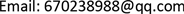﻿ 时标上一类二维动力系统的非振荡解的存在性 Existence of Nonoscillatory Solutions of Two-Dimensional Time Scale Systems

Vol. 08  No. 12 ( 2019 ), Article ID: 33381 , 8 pages
10.12677/AAM.2019.812226

Existence of Nonoscillatory Solutions of Two-Dimensional Time Scale Systems

Ting Li, Feng Chen

School of Mathematics and Information Science, Guangxi University, Nanning GuangxiReceived: Nov. 15th, 2019; accepted: Dec. 3rd, 2019; published: Dec. 10th, 2019ABSTRACT

The study of time-scale theory combines the theory of differential equations with the theory of difference equations effectively, and the results obtained are more extensively used than those of differential equations and difference equations. In this paper, we employ Schauder’s fixed point theorem, Knaster’s fixed point theorem and double improper integrals to establish the existence of nonoscillatory solutions to the two-dimensional time scale system composed of first-order dynamic equations, and verify the main obtained results through some examples.

Keywords:Two-Dimensional System, Nonoscillatory Solutions, Time Scales, Existence1. 引言

$\left\{\begin{array}{l}{x}^{\Delta }\left(t\right)=f\left(t,y\left(t\right)\right)\\ {y}^{\Delta }\left(t\right)=g\left(t,x\left(t\right)\right)\end{array}$ (1.1)

$\left\{\begin{array}{l}{x}^{\prime }\left(t\right)=a\left(t\right)f\left(y\left(t\right)\right)\\ {y}^{\prime }\left(t\right)=b\left(t\right)g\left(x\left(t\right)\right)\end{array}$ (1.2)

$\left\{\begin{array}{l}{x}^{\Delta }\left(t\right)=a\left(t\right)f\left(y\left(t\right)\right)\\ {y}^{\Delta }\left(t\right)=b\left(t\right)g\left(z\left(t\right)\right)\\ {z}^{\Delta }\left(t\right)=c\left(t\right)h\left(x\left(t\right)\right)\end{array}$ (1.3)

2. 几个引理

$\begin{array}{l}{S}^{+}:=\left\{\left(x,y\right)\in S:\underset{t\to \infty }{\mathrm{lim}}x\left(t\right)y\left(t\right)>0\right\}\\ {S}^{-}:=\left\{\left(x,y\right)\in S:\underset{t\to \infty }{\mathrm{lim}}x\left(t\right)y\left(t\right)<0\right\}\end{array}$

$\begin{array}{l}{S}_{B,B}^{+}:=\left\{\left(x,y\right)\in {S}^{+}:\underset{t\to \infty }{\mathrm{lim}}x\left(t\right)=c,\underset{t\to \infty }{\mathrm{lim}}y\left(t\right)=d\right\}\\ {S}_{B,\infty }^{+}:=\left\{\left(x,y\right)\in {S}^{+}:\underset{t\to \infty }{\mathrm{lim}}x\left(t\right)=c,\underset{t\to \infty }{\mathrm{lim}}y\left(t\right)=\infty \right\}\end{array}$

$\begin{array}{l}{S}_{\infty ,B}^{+}:=\left\{\left(x,y\right)\in {S}^{+}:\underset{t\to \infty }{\mathrm{lim}}x\left(t\right)=\infty ,\underset{t\to \infty }{\mathrm{lim}}y\left(t\right)=d\right\}\\ {S}_{\infty ,\infty }^{+}:=\left\{\left(x,y\right)\in {S}^{+}:\underset{t\to \infty }{\mathrm{lim}}x\left(t\right)=\infty ,\underset{t\to \infty }{\mathrm{lim}}y\left(t\right)=\infty \right\}\end{array}$

3. 主要结论

${\int }_{{t}_{0}}^{\infty }f\left(s,L+{\int }_{{t}_{0}}^{s}g\left(u,{K}_{1}\right)\Delta u\right)\Delta s<\infty$ (3.1)

${\int }_{{t}_{0}}^{\infty }g\left(s,{K}_{2}\right)\Delta s<\infty$ (3.2)

$g\left(t,u\right)$ 关于u的单调性和对(1.1)的第二个方程由 ${t}_{1}$ 到t进行积分，得

$y\left(t\right)=y\left({t}_{1}\right)+{\int }_{{t}_{1}}^{t}g\left(s,x\left(s\right)\right)\Delta s\ge y\left({t}_{1}\right)+{\int }_{{t}_{1}}^{t}g\left(s,\frac{c}{2}\right)\Delta s$ (3.3)

$t\to \infty$ 时，可得

${\int }_{{t}_{1}}^{\infty }g\left(s,\frac{c}{2}\right)\Delta s\le d-y\left({t}_{1}\right)<\infty$

$c\ge x\left(t\right)\ge x\left({t}_{1}\right)+{\int }_{{t}_{1}}^{t}f\left(s,y\left({t}_{1}\right)+{\int }_{{t}_{1}}^{s}g\left(u,\frac{c}{2}\right)\Delta u\right)\Delta s$

$t\to \infty$ 时，有

${\int }_{{t}_{1}}^{t}f\left(s,y\left({t}_{1}\right)+{\int }_{{t}_{1}}^{s}g\left(u,\frac{c}{2}\right)\Delta u\right)\Delta s\le c-x\left({t}_{1}\right)<\infty$

$Y=\left\{x\left(t\right)\in X:\frac{{K}_{1}}{2}\le x\left(t\right)\le {K}_{1},t\ge {t}_{1}\right\}$

$\left(Fx\right)\left(t\right)={K}_{1}-{\int }_{t}^{\infty }f\left(s,L+{\int }_{{t}_{1}}^{s}g\left(u,x\left(u\right)\right)\Delta u\right)\Delta s$

1) 先证F为自映射算子，

${K}_{1}\ge \left(Fx\right)\left(t\right)\ge {K}_{1}-{\int }_{t}^{\infty }f\left(s,L+{\int }_{{t}_{1}}^{s}g\left(u,{K}_{1}\right)\Delta u\right)\Delta s\ge \frac{{K}_{1}}{2}$

2) 再证F在Y上连续，取Y上的一组函数列 ${x}_{n}$，使得 ${x}_{n}\to x$$x\in Y$

$|\left(F{x}_{n}\right)\left(t\right)-\left(Fx\right)\left(t\right)|\le {\int }_{t}^{\infty }|f\left(s,L+{\int }_{{t}_{1}}^{s}g\left(u,{x}_{n}\left(u\right)\right)\Delta u\right)-f\left(s,L+{\int }_{{t}_{1}}^{s}g\left(u,x\left(u\right)\right)\Delta u\right)|\Delta s$

3) 最后证明FY相对紧致，由于

$0<{\left(Fx\right)}^{\Delta }\left(t\right)\le f\left(t,L+{\int }_{{t}_{1}}^{t}g\left(u,{K}_{1}\right)\Delta u\right)<\infty$

$\stackrel{¯}{x}\left(t\right)={K}_{1}-{\int }_{t}^{\infty }f\left(s,L+{\int }_{{t}_{1}}^{s}g\left(u,\stackrel{¯}{x}\left(u\right)\right)\Delta u\right)\Delta s$

${\stackrel{¯}{x}}^{\Delta }\left(t\right)=f\left(t,L+{\int }_{{t}_{1}}^{t}g\left(u,\stackrel{¯}{x}\left(u\right)\right)\Delta u\right)$

$\stackrel{¯}{y}\left(t\right)=L+{\int }_{{t}_{1}}^{t}g\left(u,\stackrel{¯}{x}\left(u\right)\right)\Delta u$

$t\to \infty$ 时，有 $\stackrel{¯}{x}\left(t\right)\to {K}_{1}$$\stackrel{¯}{y}\left(t\right)\to \beta$，其中 $0<{K}_{1},\beta <\infty$，故 $\left(\stackrel{¯}{x},\stackrel{¯}{y}\right)$ 是(1.1)的非振荡，因此 ${S}_{B,B}^{+}\ne \varnothing$，证毕。

${\int }_{{t}_{0}}^{\infty }f\left(s,{\int }_{{t}_{0}}^{s}g\left(u,{K}_{1}\right)\Delta u\right)\Delta s<\infty$ (3.4)

${\int }_{{t}_{0}}^{\infty }g\left(s,{K}_{2}\right)\Delta s=\infty$ (3.5)

$\frac{c}{2}\le x\left(t\right)=x\left({t}_{1}\right)+{\int }_{{t}_{1}}^{t}f\left(s,y\left(s\right)\right)\Delta s\le c$

$t\to \infty$ 时，有

${\int }_{{t}_{1}}^{\infty }f\left(s,y\left(s\right)\right)\Delta s\le c-x\left({t}_{1}\right)<\infty$ (3.6)

$g\left(t,u\right)$ 关于u的单调性和对(1.1)的第二个方程由 ${t}_{1}$ 到t进行积分，得

$y\left({t}_{1}\right)+{\int }_{{t}_{1}}^{t}g\left(s,c\right)\Delta s\ge y\left(t\right)=y\left({t}_{1}\right)+{\int }_{{t}_{1}}^{t}g\left(s,x\left(s\right)\right)\Delta s$

$t\to \infty$ 时，有

${\int }_{{t}_{1}}^{\infty }g\left(s,c\right)\Delta s=\infty$ (3.7)

${\int }_{{t}_{1}}^{\infty }f\left(s,{\int }_{{t}_{1}}^{s}g\left(u,\frac{c}{2}\right)\Delta u\right)\Delta s\le {\int }_{{t}_{1}}^{\infty }f\left(s,y\left(s\right)\right)\Delta s<\infty$

$\Omega =\left\{x\left(t\right)\in X:K\le x\left(t\right)\le 2K,t\ge {t}_{1}\right\}$

$\left(Fx\right)\left(t\right)=K+{\int }_{{t}_{1}}^{t}f\left(s,{\int }_{{t}_{1}}^{s}g\left(u,x\left(u\right)\right)\Delta u\right)\Delta s$

$K\le \left(Fx\right)\left(t\right)\le K+{\int }_{{t}_{1}}^{t}f\left(s,{\int }_{{t}_{1}}^{s}g\left(u,2K\right)\Delta u\right)\Delta s\le 2K$

$\stackrel{¯}{x}\left(t\right)=K+{\int }_{{t}_{1}}^{t}f\left(s,{\int }_{{t}_{1}}^{s}g\left(u,\stackrel{¯}{x}\left(u\right)\right)\Delta u\right)\Delta s$

${\stackrel{¯}{x}}^{\Delta }\left(t\right)=f\left(t,{\int }_{{t}_{1}}^{t}g\left(u,\stackrel{¯}{x}\left(u\right)\right)\Delta u\right)$

$\stackrel{¯}{y}\left(t\right)={\int }_{{t}_{1}}^{t}g\left(u,\stackrel{¯}{x}\left(u\right)\right)\Delta u$

$t\to \infty$ 时，有 $\stackrel{¯}{x}\left(t\right)\to \alpha$$\stackrel{¯}{y}\left(t\right)\to \infty$，其中 $0<\alpha <\infty$，故 $\left(\stackrel{¯}{x},\stackrel{¯}{y}\right)$ 是(1.1)的非振荡解，因此 ${S}_{B,\infty }^{+}\ne \varnothing$，证毕。

${\int }_{{t}_{0}}^{\infty }g\left(s,{\int }_{{t}_{0}}^{s}f\left(u,{K}_{1}\right)\Delta u\right)\Delta s<\infty$

${\int }_{{t}_{0}}^{\infty }f\left(s,{K}_{2}\right)\Delta s=\infty$

4. 例子

$\left\{\begin{array}{l}{x}^{\Delta }\left(t\right)=\frac{q+1}{{q}^{2}{t}^{3}}{e}_{\frac{ty\left(t\right)-t+1}{\left(q-1\right){t}^{2}+\left(2-q\right)t}}\left(t,1\right),\\ {y}^{\Delta }\left(t\right)=\frac{1}{q\left(2{t}^{2}-1\right)}x\left(t\right).\end{array}$ (4.1)

$\begin{array}{c}{\int }_{1}^{\infty }g\left(t,K\right)\Delta t={\int }_{1}^{\infty }\frac{K}{q\left(2{t}^{2}-1\right)}\Delta t\\ =\frac{K}{q}\underset{t\in {\left[1,\infty \right)}_{{q}^{{ℕ}_{0}}}}{\sum }\frac{1}{2{t}^{2}-1}\left(q-1\right)t\le \text{}\frac{\left(q-1\right)K}{q}\underset{t\in {\left[1,\infty \right)}_{{q}^{{ℕ}_{0}}}}{\sum }\frac{1}{t}\text{}\\ =\frac{\left(q-1\right)K}{q}\underset{t\in {\left[1,\infty \right)}_{{q}^{{ℕ}_{0}}}}{\sum }\frac{1}{{q}^{n}}=K<\infty \end{array}$

$\begin{array}{l}{\int }_{1}^{\infty }\frac{q+1}{{q}^{2}{t}^{3}}f\left(t,\frac{1}{2}+{\int }_{1}^{t}\frac{1}{q\left(2{t}^{2}-1\right)}g\left(u,\frac{1}{2}\right)\Delta u\right)\Delta t\le {\int }_{1}^{\infty }\frac{q+1}{{q}^{2}{t}^{3}}f\left(t,1\right)\Delta t\\ ={\int }_{1}^{\infty }\frac{q+1}{{q}^{2}{t}^{3}}{e}_{\frac{1}{t+\left(q-1\right)\left(t-1\right)t}}\left(t,1\right)\Delta t\\ =\underset{t\in {\left[1,\infty \right)}_{{q}^{{ℕ}_{0}}}}{\sum }\frac{q+1}{{q}^{2}{t}^{3}}{e}_{\frac{1}{t+\left(q-1\right)\left(t-1\right)t}}\left(t,1\right)\left(q-1\right)t\\ =\frac{{q}^{2}-1}{{q}^{2}}\underset{t\in {\left[1,\infty \right)}_{{q}^{{ℕ}_{0}}}}{\sum }\frac{1}{{t}^{2}}\mathrm{exp}\left\{\frac{1}{\left(q-1\right)s}{\int }_{1}^{t}\mathrm{ln}\left(1+\left(q-1\right)s\right)\frac{\frac{1}{s}}{1+\left(q-1\right)\left(s-1\right)s}\Delta s\right\}\end{array}$

$\begin{array}{l}<\frac{{q}^{2}-1}{{q}^{2}}\underset{t\in {\left[1,\infty \right)}_{{q}^{{ℕ}_{0}}}}{\sum }\frac{1}{{t}^{2}}\mathrm{exp}\left\{\mathrm{ln}q{\int }_{1}^{t}\frac{1}{\left(q-1\right)s}\Delta s\right\}\\ =\frac{{q}^{2}-1}{{q}^{2}}\underset{t\in {\left[1,\infty \right)}_{{q}^{{ℕ}_{0}}}}{\sum }\frac{1}{{t}^{2}}\mathrm{exp}\left\{\mathrm{ln}q\underset{m=0}{\overset{n}{\sum }}1\right\}\\ =\frac{{q}^{2}-1}{{q}^{2}}\underset{t\in {\left[1,\infty \right)}_{{q}^{{ℕ}_{0}}}}{\sum }\frac{1}{t}\\ =\frac{{q}^{2}-1}{{q}^{2}}\underset{n=0}{\overset{\infty }{\sum }}\frac{1}{{q}^{n}}=\frac{q+1}{q}<\infty \end{array}$

$\left\{\begin{array}{l}{x}^{\Delta }\left(t\right)=\frac{t+{\left(\sqrt{t}+1\right)}^{2}}{t\left({t}^{2}+1\right)\left[{\left(\sqrt{t}+1\right)}^{4}+1\right]}y\left(t\right),\\ {y}^{\Delta }\left(t\right)={e}_{\frac{\left(x\left(t\right)-1\right)\left({t}^{2}+1\right)+1}{{t}^{2}+1+{t}^{2}\left(1+2\sqrt{t}\right)}}\left(t,1\right).\end{array}$ (4.2)

$\begin{array}{c}{\int }_{1}^{\infty }g\left(t,1\right)\Delta t={\int }_{1}^{\infty }\frac{{e}_{1}\left(t,1\right)}{{e}_{1-\frac{1}{{t}^{2}+1}}\left(t,1\right)}\Delta t\\ ={\int }_{1}^{\infty }{e}_{\frac{\frac{1}{{t}^{2}+1}}{1+\left(1+2\sqrt{t}\right)\left(1-\frac{1}{{t}^{2}+1}\right)}}\left(t,1\right)\Delta t\\ \ge \text{}{\int }_{1}^{\infty }\mathrm{exp}\left\{{\int }_{1}^{t}\frac{1}{1+2\sqrt{s}}\mathrm{ln}\left(1\right)\Delta s\right\}\Delta t=\infty \end{array}$

$\begin{array}{c}{\int }_{1}^{\infty }f\left(t,{\int }_{1}^{t}g\left(u,1\right)\Delta u\right)\Delta t\\ ={\int }_{1}^{\infty }\frac{t+{\left(\sqrt{t}+1\right)}^{2}}{t\left({t}^{2}+1\right)\left[{\left(\sqrt{t}+1\right)}^{4}+1\right]}\left({\int }_{1}^{t}\frac{{e}_{1}\left(u,1\right)}{{e}_{1-\frac{1}{{u}^{2}+1}}\left(u,1\right)}\Delta u\right)\Delta t\\ ={\int }_{1}^{\infty }\frac{t+{\left(\sqrt{t}+1\right)}^{2}}{t\left({t}^{2}+1\right)\left[{\left(\sqrt{t}+1\right)}^{4}+1\right]}\left({\int }_{1}^{t}{e}_{\frac{1}{{u}^{2}+1+{u}^{2}\left(1+2\sqrt{u}\right)}}\left(u,1\right)\Delta u\right)\Delta t\\ ={\int }_{1}^{\infty }\frac{t+{\left(\sqrt{t}+1\right)}^{2}}{t\left({t}^{2}+1\right)\left[{\left(\sqrt{t}+1\right)}^{4}+1\right]}\left({\int }_{1}^{t}\mathrm{exp}\left\{{\int }_{1}^{u}\frac{1}{1+2\sqrt{s}}\mathrm{ln}\left(2+2\sqrt{s}\right)\frac{1}{{s}^{2}+1+{s}^{2}\left(1+2s\right)}\Delta s\right\}\Delta u\right)\Delta t\end{array}$

$\begin{array}{c}\le {\int }_{1}^{\infty }\frac{t+{\left(\sqrt{t}+1\right)}^{2}}{t\left({t}^{2}+1\right)\left[{\left(\sqrt{t}+1\right)}^{4}+1\right]}\left({\int }_{1}^{t}u\Delta u\right)\Delta t\\ \le {\int }_{1}^{\infty }\frac{t+{\left(\sqrt{t}+1\right)}^{2}}{t\left({t}^{2}+1\right)\left[{\left(\sqrt{t}+1\right)}^{4}+1\right]}{t}^{2}\Delta t\\ \le {\int }_{1}^{\infty }\frac{1}{t{\left(\sqrt{t}+1\right)}^{2}}\frac{t+{\left(\sqrt{t}+1\right)}^{2}}{{\left(\sqrt{t}+1\right)}^{2}}\Delta t\\ \le {\int }_{1}^{\infty }\frac{2}{t\sigma \left(t\right)}\Delta t=2<\infty \end{array}$

Existence of Nonoscillatory Solutions of Two-Dimensional Time Scale Systems[J]. 应用数学进展, 2019, 08(12): 1971-1978. https://doi.org/10.12677/AAM.2019.812226

1. 1. Li, W.T. (2002) Classification Schemes for Positive Solutions of Nonlinear Differential Systems. Mathematical and Computer Modeling, 36, 411-418.
https://doi.org/10.1016/S0895-7177(02)00172-3

2. 2. Öztürk, Ö. (2017) On the Existence of Nonoscillatory Solutions of Three-Dimensional Time Scale Systems. Fixed Point Theory and Applications, 19, 2617-2628.
https://doi.org/10.1007/s11784-017-0454-9

3. 3. Zhu, Z. and Wang, Q. (2007) Existence of Nonoscillatory Solutions to Neutral Dynamic Equations on Time Scales. Journal of Mathematical Analysis and Applications, 335, 751-762.
https://doi.org/10.1016/j.jmaa.2007.02.008

4. 4. Öztürk, Ö. (2018) On Oscillatory Behavior of Two-Dimensional Time Scale Systems. Advances in Difference Equations, 2018, 18.
https://doi.org/10.1186/s13662-018-1475-4

5. 5. Öztürk, Ö. and Akın, E. (2016) On Nonoscillatory Solutions of Two Dimensional Nonlinear Delay Dynamical Systems. Opuscula Mathematica, 36, 651-669.
https://doi.org/10.7494/OpMath.2016.36.5.651

6. 6. Öztürk, Ö. and Akın, E. (2016) Nonoscillation Criteria for Two-Dimensional Time-Scale Systems. Nonautonomous Dynamical Systems, 3, 1-13.
https://doi.org/10.1515/msds-2016-0001

7. 7. Öztürk, Ö. and Akın, E. (2016) Classification of Nonoscillatory Solutions of Nonlinear Dynamic Equations on Time Scales. Dynamic Systems and Applications, 25, 219-236.

8. 8. Bohner, M. and Peterson, A. (2001) Dynamic Equations on Time Scales. An Introduction with Applications. Birkhäuser, Boston.
https://doi.org/10.1007/978-1-4612-0201-1

9. 9. Zeidler, E. (1986) Nonlinear Functional Analysis and Its Applications I: Fixed Point Theorems. Springer, New York.
https://doi.org/10.1007/978-1-4612-4838-5

10. 10. Knaster, B. (1928) Un théorème sur les fonctions d’ensembles. Annales Societatis Polonici Mathmatici, 6, 133-134.